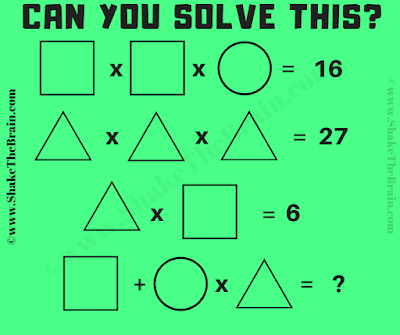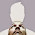## Wednesday, April 17, 2019

This is a fun shapes math picture puzzle. In this math picture puzzle, you are shown some mathematical equations. Variables in these equations are represented by geometrical shapes. Your challenge is to solve these equations and find the value of Triangle, Circle and Squares. Once you are able to find the value of these shapes, calculate the equation to find the value of the missing number which will replace the question mark.Can you solve this math puzzle?
Answer of this "Shapes Math Picture Puzzle", can be viewed by clicking on the answer button.

1.1.2.3.